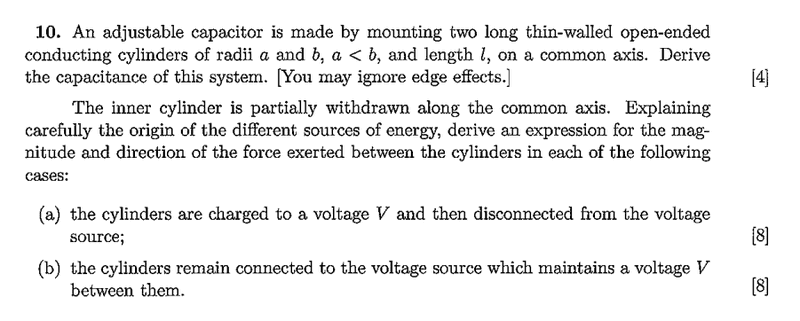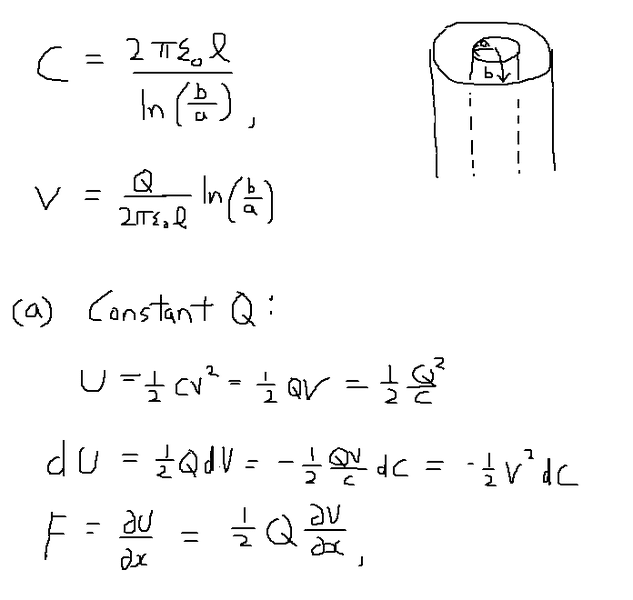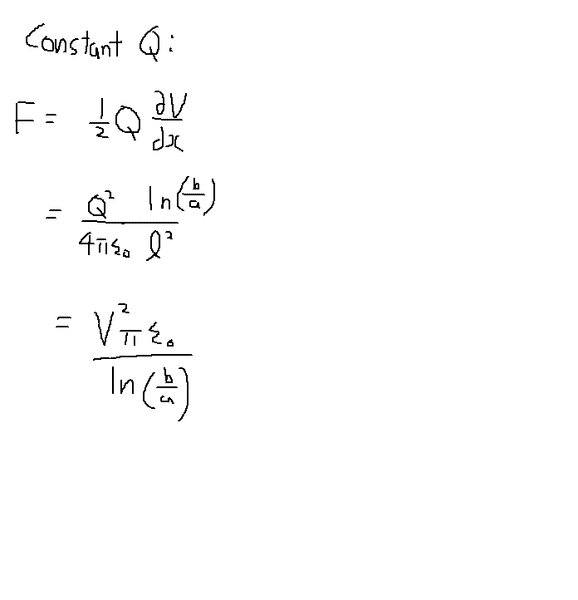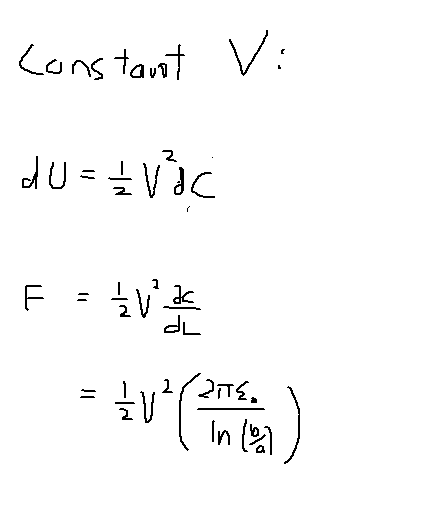# Force between cylindrical capacitors

## Homework Statement## The Attempt at a SolutionI'm not sure what is being varied here, radial separation between capacitors x = (b-a) or whether the capacitors can slide up and down so as to change the length L of the capacitor..

bumpp

TSny
Homework Helper
Gold Member
I'm not sure what is being varied here, radial separation between capacitors x = (b-a) or whether the capacitors can slide up and down so as to change the length L of the capacitor..

Since the question states that the inner cylinder is partially withdrawn along the common axis, I think it's L that is varied.

Since the question states that the inner cylinder is partially withdrawn along the common axis, I think it's L that is varied.

OK, so for constant Q, the force is (1/2)Qo(∂V/∂L), and constant V, the force is (0.5)V^2 (∂C/∂L):Last edited:
TSny
Homework Helper
Gold Member
How are you defining x? Is it the amount by which the inner conductor is withdrawn or the amount by which the inner conductor is still inside the outer conductor? Or does x represent something else?

How would you write C in terms of x?

Won't it be much better to use the equation ##U=\frac{Q^2}{2C}## and calculate the force when the charge is constant?

How are you defining x? Is it the amount by which the inner conductor is withdrawn or the amount by which the inner conductor is still inside the outer conductor? Or does x represent something else?

How would you write C in terms of x?

Sorry i meant L instead of x.

Won't it be much better to use the equation ##U=\frac{Q^2}{2C}## and calculate the force when the charge is constant?

The C is in the denominator, which gives a C-2(∂C/∂L) term